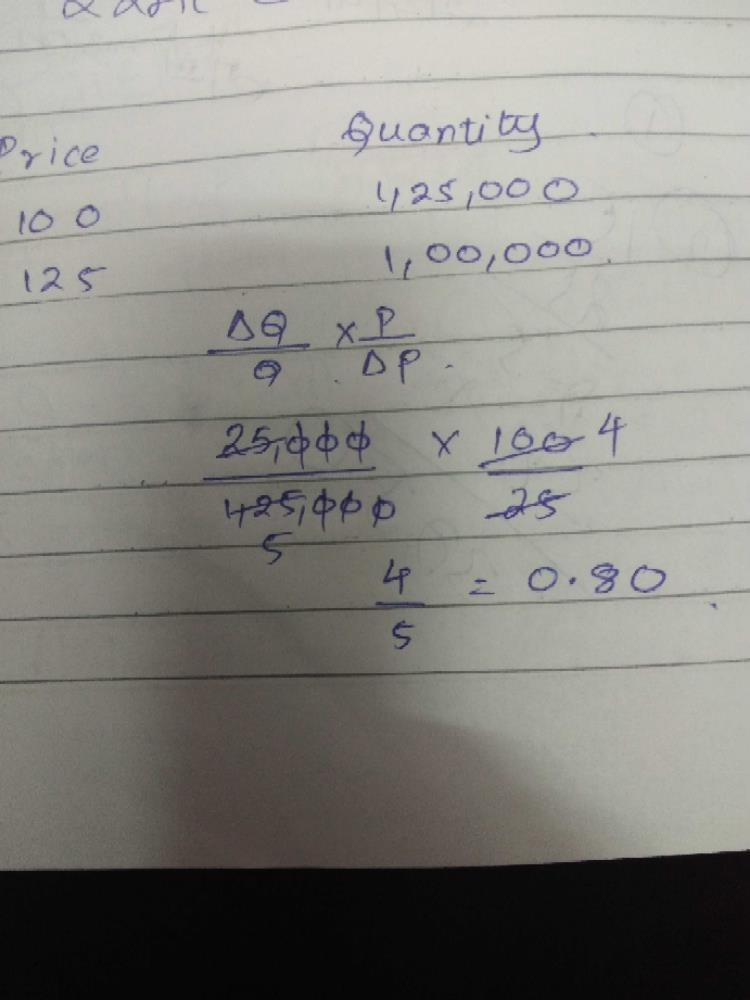Courses

# Can you explain the answer of this question below:... moreThe Price of a tiffin box is Rs. 100 per unit andthe quantity demanded in a market is 1,25,000units . Company increased the price to Rs.125per unit due to this increase in price quantitydemanded decreases to 1,00,000 units. whatwill be price elasticity of demand ______A:1.25B:1.00C:0.80D:None of the above.The answer is c. Related Test: Test: Theory Of Demand- 2

## CA Foundation QuestionSamiksha Jun 11, 2018
Well lets solve this step by step..... Given:- Q=125000 units, Q1=100000 units, change in quantity= - 25000 units, now %change in quantity demand = change in quantity /Q * 100= - 20% .. Now P=Rs 100, P1=Rs 125, change in price=Rs 25, %change in price = change in price/P * 100= 25%... nw price elasticity of demand = %change in quantity demanded /%change in price =-20%/25%=(-)0.8... i think 0.8 will be the answer plz check it once more...Naveen Garg Jun 15, 2018
Ans is one As {(125000 - 100000)/(125000+100000)} X {(125+100)/(125-100)} Solve this u get ur ans...Girish Agarwal Jun 16, 2018B. 0.80Goplar Ajay Dec 21, 2018Kirti Khandelwal May 12, 2019
0.8 ans.Swati Tevathiya May 18, 2019
0.80 right ans